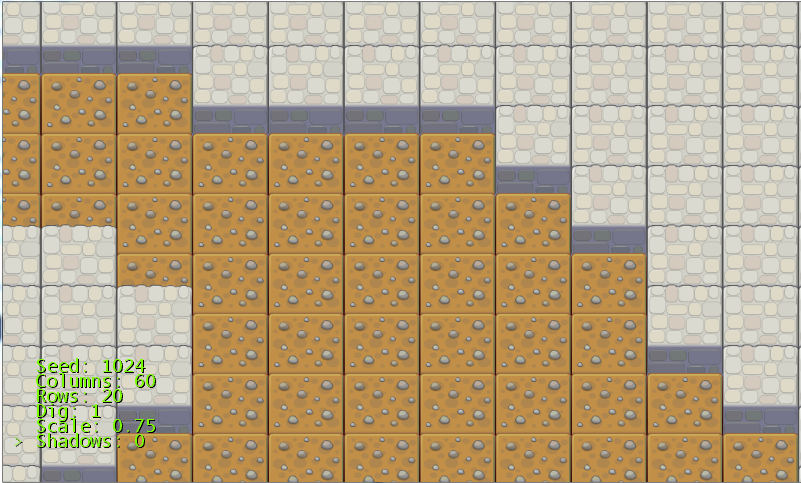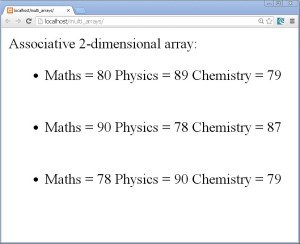Home Web Programming Tutorials Multi-Dimensional Arrays in PHP

# Multi-Dimensional Arrays in PHP

1
35453Today we are going to learn the manipulation of multi-dimensional arrays in this Multi-Dimensional Arrays in PHP.

• Arrays are used to store more than one similar type values in a single variable.
• These values can be anything like strings, integers, floats, objects and even other arrays.
• When we include arrays in array, we deal with multi-dimensional arrays.
• There are situations when we need to store multiple values for an entity and group such entities at one place. I mean we can store the values such as name, quantity and price for different products. Multi-dimensional arrays are better storages in such situations.
• We can have any number of dimensions in multi-dimensional arrays.
• Two-dimensional and Three dimensional arrays are mostly used. We can even have four, five, and six up to n dimensional arrays.
• The dimensions of an array indicate the number of indices required to fetch a value from the array. Such as two dimensional arrays will require 2 dimensions and three dimensional arrays will require 3 dimensions.
• Multi-dimensional arrays can be indexed as well as associative arrays.
• Let us go through various examples of multi-dimensional arrays:

Two-dimensional arrays:

• A two dimensional array means array of arrays.
• Example of indexed two dimensional array:
```<?php
\$marks=array(
array(80,89,79),
array(90,78,87),
array(78,90,78)
);
?>
```
• Here, we have declared a \$marks two-dimensional array since it has 3 arrays inside an array.
• This is an indexed array because the arrays will be given indexes 0, 1 and 2 automatically.
• And in turn the values inside the arrays will also be given indices starting from 0 each.
• Since it is a 2-dimensional array, it requires 2 dimensions i.e. row and column to retrieve the values.
• Let is demonstrate it by writing a code.
• To demonstrate it create a new folder named multi_arrays in the htdocs folder in the xampp folder in C drive. Open a new notepad++ document and save it as index.php in this newly created multi_arrays folder
• Write the following code in index.php file:
```<?php

echo "Indexed 2-dimensional array:<br>";
\$marks=array(
array(80,89,79),
array(90,78,87),
array(78,90,78)
);

//displaying array elements manually
echo "<strong>marks array displayed manually:</strong><br>";
echo \$marks."  ".\$marks."  ".\$marks."<br>";
echo \$marks."  ".\$marks."  ".\$marks."<br>";
echo \$marks."  ".\$marks."  ".\$marks."<br><br>";

//displaying array elements at once using for loop.
echo "<b>marks array displayed using nested for loop:</b><br>";
for(\$r=0;\$r<count(\$marks);\$r++)
{
for(\$c=0;\$c<count(\$marks[\$r]);\$c++)
{
echo \$marks[\$r][\$c]."   ";
}
echo "<br>";
}
```
• In the above code we have declared a \$marks array containing marks of 3 students in 3 subjects each.
• These elements can be accessed using 2 dimensions, one for row and other for column.
• The 3 arrays in the \$marks array are indexed with 0, 1 and 2. These will be considered as rows.
• The elements inside each row are indexed starting with 0 (zero) each. These are considered as columns.
• So the first element in first row can be accessed as \$marks, second element in first row can be accessed as \$marks and so on.
• The code for accessing all elements manually is given below:
```echo "<strong>marks array displayed manually:</strong><br>";
echo \$marks."  ".\$marks."  ".\$marks."<br>";
echo \$marks."  ".\$marks."  ".\$marks."<br>";
echo \$marks."  ".\$marks."  ".\$marks."<br><br>";
```
• The elements are accessed according to their indexes in each row and column.
• If there is a big 2-dimensional array containing large number of elements, it is not possible to access such a large number of elements manually.
• Thus we can use a nested for loop for this purpose. Why nested only, because we have more than one indices in case of multi-dimensional arrays.
• If there are 2 indices then 2 for loops, if there are 3 indices then 3 for loops and so on.
• Let us see how to access array values using loops. The code is given below:
```echo "<b>marks array displayed using nested for loop:</b><br>";
for(\$r=0;\$r<count(\$marks);\$r++)
{
for(\$c=0;\$c<count(\$marks[\$r]);\$c++)
{
echo \$marks[\$r][\$c]."   ";
}
echo "<br>";
}
```
• Here, we have used the outer for loop for row and inner for loop for column.
• The variable \$r is the counter for row for loop and \$c is the counter for column for loop.
• \$r is first initialized to 0 (zero) i.e. zeroth row.
• The condition given is
`\$r<count(\$marks)`

. Here, the function count() will count the number of arrays present in the array \$marks and return that number. In our case this condition is equal to \$r<3, because we have 3 arrays in \$marks array.

• In the inner for loop. \$c is initialized to 0 (zero) i.e zeroth column.
• The condition given here is
`\$c<count(\$marks[\$r])`

. Here, the parameter in count() function is \$marks[\$r], this indicates the particular row in the \$marks array. All together it means that the count function will count the number of elements in the particular row given. For example if the value of \$r is 0, it will count the number of elements in zeroth row and return that number. So if \$r has value 0, the condition will be like \$c<3. This is because the zeroth row contains 3 elements/columns.

• So all the elements are displayed using echo function accessing the indices using \$r and \$c variables.
• The statement is shown below:
`echo \$marks[\$r][\$c]."   ";`
• A space is left after every element in a row.
• After completion of one row a break statement is executed to leave a line break and start a new row on new line.
• The output is shown below:

• Example of associative two dimensional array:
• The same array in indexed form given above can be written in associative form as shown below:
```\$marks1=array(
array("Maths"=>80,"Physics"=>89,"Chemistry"=>79),
array("Maths"=>90,"Physics"=>78,"Chemistry"=>87),
array("Maths"=>78,"Physics"=>90,"Chemistry"=>79)
);
```
• It is similar to indexed array, but only each value is given a key for it like Maths, Physics and Chemistry.
• Let us access all the elements using for loop. But we have an associative array which is accessed using a foreach loop. So let us see the code given below which is written in index.php file:
```echo "Associative 2-dimensional array:<br>";

\$marks1=array(
array("Maths"=>80,"Physics"=>89,"Chemistry"=>79),
array("Maths"=>90,"Physics"=>78,"Chemistry"=>87),
array("Maths"=>78,"Physics"=>90,"Chemistry"=>79)
);
echo "<ul>";
for(\$r=0;\$r<count(\$marks1);\$r++)
{
echo "<li>";
foreach(\$marks1[\$r] as \$key=>\$value)
{
echo \$key." = ".\$value."  ";
}
echo "</li>";
echo "<br><br>";
}
echo "</ul>";
```
• Here, the above associative 2-dimensional array values are displayed using a nesting of for loop and foreach loop.
• The for loop is used to loop through the number of arrays present in the \$marks1 array and foreach loop is used to loop through the key-value pairs.
• Here we now know that the counter \$r of for loop is initialized to 0 and loops till the condition doesn’t become false i.e. till all the rows are not traversed completely.
• The foreach loop is used to go through each and every key-value pair.
• It takes every row and selects each key-value pair in it as shown in the statement below:
`foreach(\$marks1[\$r] as \$key=>\$value)`
• \$marks1[\$r] selects the particular row, as is the keyword and \$key fetches the key and \$value fetches the value of that key from that particular row using \$key=>\$value.
• The values of \$key and \$value are then displayed using echo function.
• Each row is printed on separate line and the HTML unordered list is used to display the data.
• The output is shown below:• fig 2

• Three-dimensional arrays:
• A three-dimensional array has arrays in an array and the inner arrays in turn have arrays inside it.
• These 3-dimensional arrays require 3 indices to access the values stored inside it.
• A three dimensional array is characterized by its height, width and depth.
• Let us see an example of 3-dimensional array:
```\$product=array(
array(
array("Epson Printer L110",100,4500),
array("Canon Printer",100,5000),
array("HP Laptop",500,40000)
),
array(
array("Micromax Canvas Lite",200,9000),
array("Samsung Galaxy",300,15000),
array("LAVA",100,4000)
)
array(
array("Sandisk 16 GB Pendrive",500,500),
array("UPS",200,3000)
)

);
```
• Here, we have declared a \$product three dimensional array since it has 2 arrays in an array and the 2 arrays inturn have 3 arrays each.
• The array contains the product’s name, product quantity and product price.
• This will require 3 loops to access its elements.
• Let us demonstrate how to do it. The code is given below:
```echo "3-dimensional array:<br>";

\$product=array(
array(
array("Epson Printer L110",100,4500),
array("Canon Printer",100,5000),
array("HP Laptop",500,40000)
),
array(
array("Micromax Canvas Lite",200,9000),
array("Samsung Galaxy",300,15000),
array("LAVA",100,4000)
),
array(
array("Sandisk 16 GB Pendrive",500,500),
array("UPS",200,3000)
)
);

// accessing elements from 3-dimensional product array.
echo "<tr>";
echo "<th>Product</th>";
echo "<th>Quantity</th>";
echo "<th>Price</th>";
echo "</tr>";

for(\$l=0;\$l<3;\$l++)
{
echo "<tr>";
for(\$r=0;\$r<3;\$r++)
{

for(\$c=0;\$c<3;\$c++)
{
echo "<td>".\$product[\$l][\$r][\$c]."</td>";
}
echo "</tr>";

}

}
echo "</table>";
```
• In the above code we have used 3 for loops to display the \$product array content.
• The values are displayed in a HTML table.
• The outer for loop is used for the layer. You imagine a three dimensional array as layers of 2-dimensional arrays one above the other. We consider this layer in the first for loop.
• Next the second for loop is used for rows and the inner most for loop is used for columns.
• The value is displayed using echo function as shown below:
`echo "<td>".\$product[\$l][\$r][\$c]."</td>";`
• The output of the above code is shown below:

• In the similar way arrays with multiple dimensions like four, five etc. can also be used. But mostly in the applications multi-dimensional arrays with 2 and 3 dimensions are enough.

Thus we finished learning how to make use of multi-dimensional arrays in this Multi-dimensional arrays in PHP.

#### 1 COMMENT

1.Mr.Nice

Nice